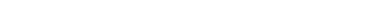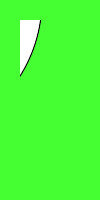# Wand chop() function – Python

The chop() function is an inbuilt function in the Python Wand ImageMagick library which is used to remove a region of an image.

Syntax:

`chop(width, height, x, y)`

Parameters: This function accepts four parameters as mentioned above and defined below:

• width: This parameter stores the size of the region.
• height: This parameter stores the size of the region.
• x: This parameter stores the x-offset of the image.
• y: This parameter stores the y-offset of the image.

Return Value: This function returns the Wand ImageMagick object.

Original Image:Example 1:

## Python3

 `# Import library from Image ` `from` `wand.image ``import` `Image`   `# Import the image` `with Image(filename ``=``'../geeksforgeeks.png'``) as image:` `    ``# Clone the image in order to process` `    ``with image.clone() as chop:` `        ``# Invoke chop function ` `        ``chop.chop(``300``, ``200``, ``15``, ``15``)` `        ``# Save the image` `        ``chop.save(filename ``=``'chop1.jpg'``)`

Output:Example 2:

## Python3

 `# Import libraries from the wand  ` `from` `wand.image ``import` `Image` `from` `wand.drawing ``import` `Drawing` `from` `wand.color ``import` `Color`   `with Drawing() as draw:` `    ``# Set Stroke color the circle to black` `    ``draw.stroke_color ``=` `Color(``'black'``)` `    ``# Set Width of the circle to 2 ` `    ``draw.stroke_width ``=` `1` `    ``# Set the fill color to 'White (# FFFFFF)'` `    ``draw.fill_color ``=` `Color(``'white'``)`   `    ``# Invoke Circle function with center at 50, 50 and radius 25` `    ``draw.circle((``200``, ``200``), ``# Center point` `                ``(``100``, ``100``)) ``# Perimeter point` `    ``# Set the font style` `    ``draw.font ``=` `'../Helvetica.ttf'` `    ``# Set the font size ` `    ``draw.font_size ``=` `30` `    `  `    ``with Image(width ``=` `400``, height ``=` `400``, background ``=` `Color(``'# 45ff33'``)) as pic:` `        ``# Set the text and its location` `        ``draw.text(``int``(pic.width ``/` `3``), ``int``(pic.height ``/` `2``), ``'GeeksForGeeks !'``)` `        ``# Draw the picture` `        ``draw(pic)` `        ``# Invoke chop function` `        ``pic.chop(``300``, ``200``, ``20``, ``20``)` `        ``# Save the image ` `        ``pic.save(filename ``=``'chop2.jpg'``)`

Output:Whether you're preparing for your first job interview or aiming to upskill in this ever-evolving tech landscape, GeeksforGeeks Courses are your key to success. We provide top-quality content at affordable prices, all geared towards accelerating your growth in a time-bound manner. Join the millions we've already empowered, and we're here to do the same for you. Don't miss out - check it out now!

Previous
Next